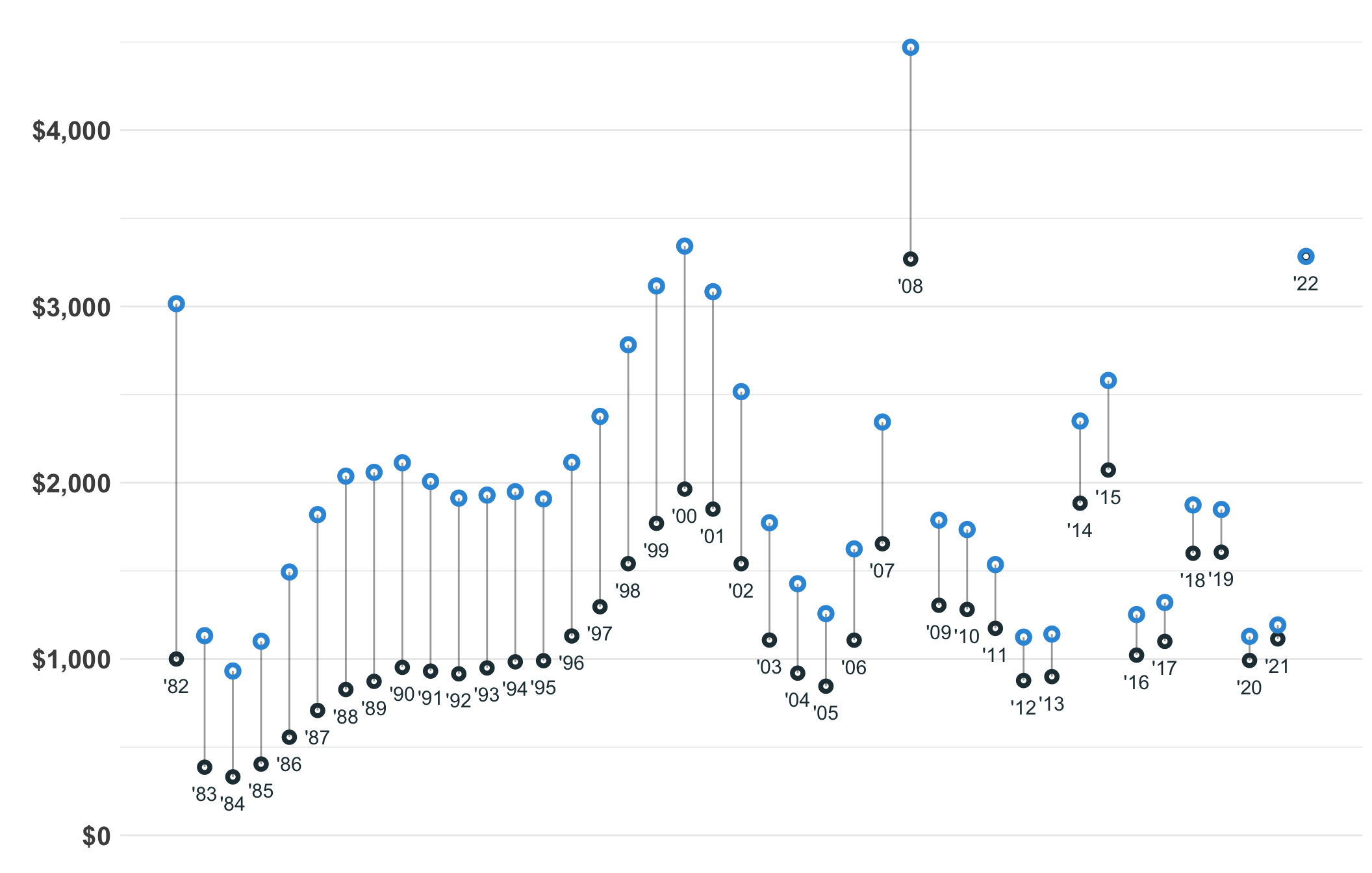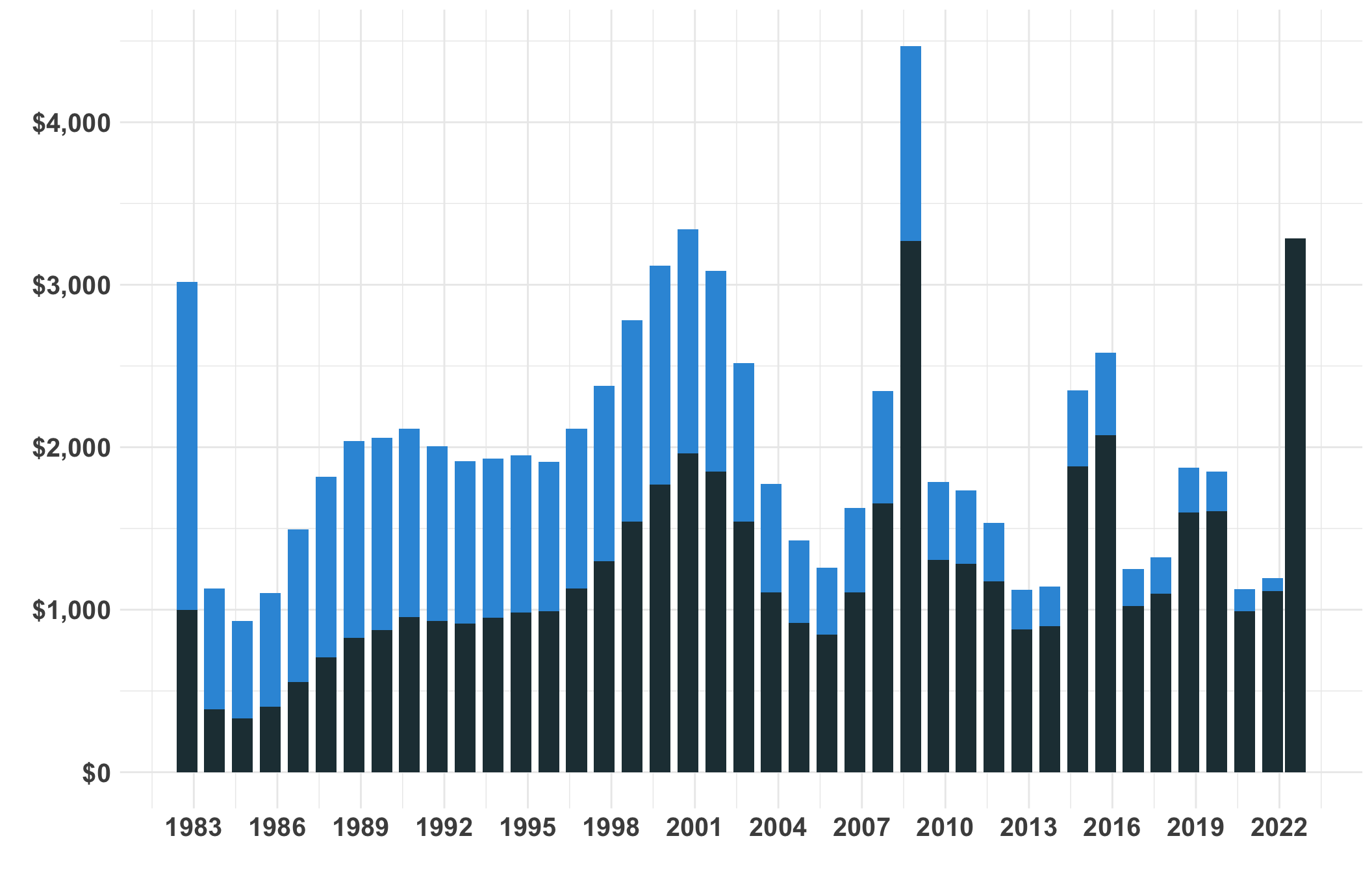Author

Ben Matheson

Published

September 20, 2022

Including the energy bonus of \$662, the 2022 Alaska Permanent Fund Dividend of \$3,284 is the largest nominal dividend in history. But the 2008 (\$4,470) and 2000 (\$3,343) dividends are larger when you compare the equivalent buying power by adjusting for inflation. See the full table below.

Six dividends are worth more than \$3,000 in 2022 dollars. And the first ever dividend in 1982 is almost as big as the 2022 record dividend. I include the energy bonus in 2008 and 2022 in the calculations here.

This page describes historic Alaska Permanent Fund Dividends are when adjusted for inflation. And I wanted to practice making more Quarto documents The code used to calculate the 2022 values is included at the bottom of this page.

The Consumer Price Index (CPI) used here is:

CPI for All Urban Consumers (CPI-U)
Series Title : All items in U.S. city average, all urban consumers, not seasonally adjusted Series ID : CUUR0000SA0
Survey Name : CPI for All Urban Consumers (CPI-U)
Measure Data Type : All items
Area : U.S. city average
Item : All items

###### BLS CPI All Urban Consumers (CPI-U)###### BLS CPI All Urban Consumers (CPI-U)###### BLS CPI All Urban Consumers (CPI-U)
Top Alaska PFDs in 2022 Dollars
Year Nominal Dividend Real (2022 Dollars) Inflation \$
2008 \$3,269 \$4,470 \$1,201
2000 \$1,964 \$3,343 \$1,379
2022 \$3,284 \$3,284 \$0
1999 \$1,770 \$3,116 \$1,347
2001 \$1,850 \$3,084 \$1,234
1982 \$1,000 \$3,016 \$2,016
1998 \$1,541 \$2,783 \$1,242
2015 \$2,072 \$2,580 \$508
2002 \$1,541 \$2,517 \$976
1997 \$1,297 \$2,376 \$1,080
2014 \$1,884 \$2,350 \$466
2007 \$1,654 \$2,345 \$691
1996 \$1,131 \$2,115 \$985
1990 \$953 \$2,113 \$1,161
1989 \$873 \$2,059 \$1,186
1988 \$827 \$2,038 \$1,211
1991 \$931 \$2,008 \$1,076
1994 \$984 \$1,949 \$965
1993 \$949 \$1,930 \$981
1992 \$916 \$1,913 \$997
1995 \$990 \$1,908 \$918
2018 \$1,600 \$1,874 \$274
2019 \$1,606 \$1,848 \$242
1987 \$708 \$1,819 \$1,111
2009 \$1,305 \$1,788 \$483
2003 \$1,108 \$1,773 \$666
2010 \$1,281 \$1,735 \$454
2006 \$1,107 \$1,625 \$518
2011 \$1,174 \$1,536 \$362
1986 \$556 \$1,494 \$937
2004 \$920 \$1,427 \$507
2017 \$1,100 \$1,321 \$221
2005 \$846 \$1,257 \$412
2016 \$1,022 \$1,252 \$230
2021 \$1,114 \$1,193 \$79
2013 \$900 \$1,141 \$241
1983 \$386 \$1,132 \$746
2020 \$992 \$1,128 \$136
2012 \$878 \$1,124 \$246
1985 \$404 \$1,101 \$697
1984 \$331 \$932 \$601

## Inflation Calculation code:

Show the code
``````#| warning: false
#| message: false

###CPI SOURCE: https://www.bls.gov/cpi/
# CPI for All Urban Consumers (CPI-U) 1982-84=100 (Unadjusted) - CUUR0000SA0

# pfd_raw <- read_csv("data/20210925_pfd_amounts - Sheet1.csv")

# 2022

##2022

# https://beta.bls.gov/dataViewer/view/timeseries/CUUR0000SA0;jsessionid=91432F7B2E9664054BA15E11CE3D4AC9

# CPI for All Urban Consumers (CPI-U)
#
# Series Title  :   All items in U.S. city average, all urban consumers, not seasonally adjusted
# Series ID :   CUUR0000SA0
# Seasonality   :   Not Seasonally Adjusted
# Survey Name   :   CPI for All Urban Consumers (CPI-U)
# Measure Data Type :   All items
# Area  :   U.S. city average
# Item  :   All items

cpi_raw <- read_xlsx("data/DataFinder-20220915202809.xlsx", skip=10) ### from

pfd <- pfd_raw %>% clean_names()

pfd <- pfd %>% select(dividend_year, dividend_amount)
pfd <- pfd %>% mutate (pfd_total = str_remove_all(dividend_amount, fixed("\$")))
pfd <- pfd %>% mutate (pfd_total = str_remove_all(pfd_total, fixed(",")))
pfd <- pfd %>% mutate (pfd_total = as.numeric(pfd_total))

##############################clean up cpi######################
####################################################################
##############################3

cpi <- cpi_raw %>% clean_names()
# View(cpi)
# cpi <- cpi %>% select(year, oct, aug)
# cpi <- cpi %>% mutate(cpi_index = oct)

cpi <- cpi %>% mutate(date = ym(label))

cpi <- cpi %>% mutate (cpi_index_2022 = 296.171)

cpi <- cpi %>% mutate (cpi_ratio =  observation_value/cpi_index_2022)
cpi <- cpi %>% mutate (year = as.numeric(year))

cpi_annual <- cpi %>% filter (period == "M10" | label == "2022 Aug")

#
# cpi <- cpi %>% mutate (cpi_index = ifelse(is.na(oct), aug, oct))
# cpi <- cpi %>% mutate (cpi_index_2021 = 273.567)
# cpi <- cpi %>% mutate (cpi_ratio = cpi_index_2021/cpi_index)

pfd_cpi <- pfd %>% left_join(cpi_annual, by=c("dividend_year" = "year"))
pfd_cpi <- pfd_cpi %>% mutate (dividend_2022_dollars = dividend_amount/cpi_ratio)

# pfd_cpi <- pfd_cpi %>% mutate (pfd_amount_2021 = pfd_total*cpi_ratio)
#
# pfd_cpi1 <- pfd_cpi %>% mutate (pfd_amount_2021 = pfd_total*cpi_ratio)

##make two copoes for the plot

pfd_cpi_original <- pfd_cpi %>% mutate(time =0, pfd_amount_plot  = pfd_total)
pfd_cpi_adjusted <- pfd_cpi %>% mutate(time = 1, pfd_amount_plot = dividend_2022_dollars)

pfd_cpi <- pfd_cpi %>% mutate (inflation_addition = dividend_2022_dollars  -pfd_total)``````

## Line/dot chart code

Show the code
``````#| warning: false
#| message: false
#| fig-height: 10
#| fig-width: 11

ggplot(pfd_cpi)+
geom_line(aes(y= dividend_year, x = pfd_amount_plot, group= dividend_year ), color="#000000", alpha=.4)+

geom_point( data = pfd_cpi %>% filter (time ==0 ),aes(y= dividend_year, x=pfd_total ), color="#233B43", shape =21, stroke=2)+
geom_point(data = pfd_cpi %>% filter (time ==1), aes(y= dividend_year, x=dividend_2022_dollars ), shape=21,stroke=2, size=2, color="#3498db")+
#

geom_text( data = pfd_cpi %>% filter (time ==0 ),aes(x= pfd_total-150, y=dividend_year, label=paste0("'",  substring(as.character(dividend_year) , 3,4)    )), color="#233B43", size=4)+
# geom_text( data = pfd_cpi %>% filter (time ==0 ),aes(x= pfd_total-150, y=dividend_year, label= dividend_year   ), color="#233B43", size=3.4, angle=45)+
# geom_text(data = pfd_cpi %>% filter (time ==1), aes(y= 1, x=pfd_amount_2021, label=dividend_year ), color="#3498db")+

# ggtitle("Alaska PFD Adjusted for Inflation", "PFD amounts set to September 2022 Dollars")+
theme_minimal()+
# xlim(0,4400)+
xlab("")+
scale_y_continuous(labels = NULL, breaks = c(0,1), labs(x = ""))+
scale_x_continuous( )+
ylab("Year")+
scale_x_continuous(labels=scales::dollar_format(), breaks= seq(0, 5500, by = 1000))+
# scale_y_date( date_breaks = "4 years", date_labels = "%Y")+
coord_flip()+
theme(axis.text = element_text(size = 15, face="bold"))     ``````

## Bar chart code:

Show the code
``````ggplot(pfd_cpi %>% filter(time==1))+
# geom_col(aes(y = pfd_amount_plot , x =date, fill=time),  alpha=.9, stat="identity" )
geom_col(aes(y = dividend_2022_dollars , x =date),fill="#3498db" , alpha=1, stat="identity" )+
geom_col(aes(y = pfd_total , x =date), fill="#233B43",  alpha=1, stat="identity" )+
# geom_col(aes(y = inflation_addition , x =date), fill="#233B43",  alpha=1, stat="identity" )+

theme_minimal()+
ylab("")+
xlab("")+
# xlab("")+
# coord_flip()+
scale_x_date( date_breaks = "3 years", date_labels = "%Y")+
scale_y_continuous(labels=scales::dollar_format())+
theme(axis.text = element_text(size = 15, face="bold"))

# geom_col(aes(x=dividend_year, y = pfd_total), fill="red", alpha=.5)``````

## Table code:

Show the code
``````#| warning: false
#| message: false

pfd_cpi %>% filter (time==0) %>%  arrange (desc(dividend_2022_dollars)) %>% select(-date, -cpi_index_2022, -label, -period, -pfd_total, -time, -pfd_amount_plot, -observation_value, -cpi_ratio) %>% gt() %>%
fmt_currency(
currency = "USD",
decimals = 0
) %>%
cols_label(dividend_year = "Year",
dividend_amount = "Nominal Dividend",
dividend_2022_dollars = "Real (2022 Dollars)",

) %>%
tab_style(
locations = cells_column_labels(columns = everything()),
style     = list(
#Give a thick border below
cell_borders(sides =  "bottom", weight = px(3)),
#Make text bold
cell_text(weight = "bold")
)
) %>%
tab_options(
#Remove border between column headers and title
column_labels.border.top.width = px(3),
column_labels.border.top.color = "transparent",
#Remove border around table
table.border.top.color = "transparent",
table.border.bottom.color = "transparent",
#Reduce the height of rows
I like these callout boxes that come with Quarto. They are `fun` and `cool`.